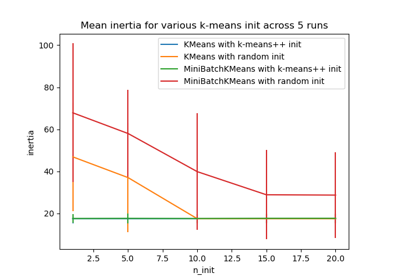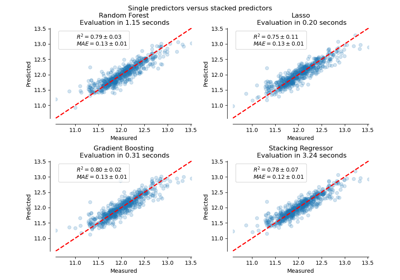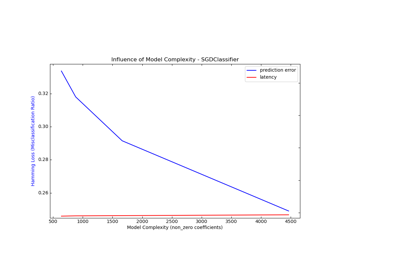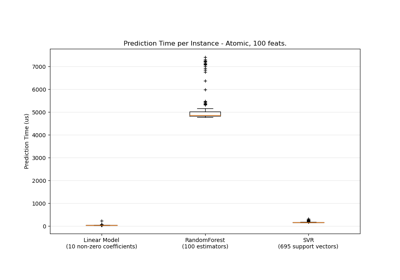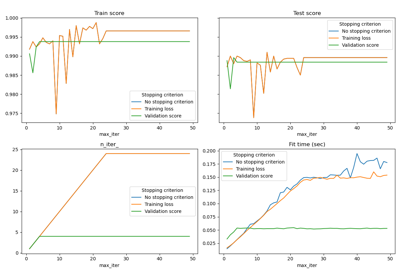# sklearn.utils.shuffle¶

sklearn.utils.shuffle(*arrays, **options)[source]

Shuffle arrays or sparse matrices in a consistent way

This is a convenience alias to resample(*arrays, replace=False) to do random permutations of the collections.

Parameters
*arrayssequence of indexable data-structures

Indexable data-structures can be arrays, lists, dataframes or scipy sparse matrices with consistent first dimension.

Returns
shuffled_arrayssequence of indexable data-structures

Sequence of shuffled copies of the collections. The original arrays are not impacted.

Other Parameters
random_stateint, RandomState instance or None, optional (default=None)

Determines random number generation for shuffling the data. Pass an int for reproducible results across multiple function calls. See Glossary.

n_samplesint, None by default

Number of samples to generate. If left to None this is automatically set to the first dimension of the arrays.

Examples

It is possible to mix sparse and dense arrays in the same run:

>>> X = np.array([[1., 0.], [2., 1.], [0., 0.]])
>>> y = np.array([0, 1, 2])

>>> from scipy.sparse import coo_matrix
>>> X_sparse = coo_matrix(X)

>>> from sklearn.utils import shuffle
>>> X, X_sparse, y = shuffle(X, X_sparse, y, random_state=0)
>>> X
array([[0., 0.],
[2., 1.],
[1., 0.]])

>>> X_sparse
<3x2 sparse matrix of type '<... 'numpy.float64'>'
with 3 stored elements in Compressed Sparse Row format>

>>> X_sparse.toarray()
array([[0., 0.],
[2., 1.],
[1., 0.]])

>>> y
array([2, 1, 0])

>>> shuffle(y, n_samples=2, random_state=0)
array([0, 1])


## Examples using sklearn.utils.shuffle¶